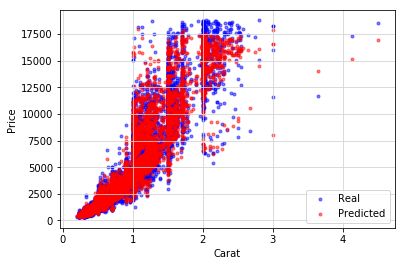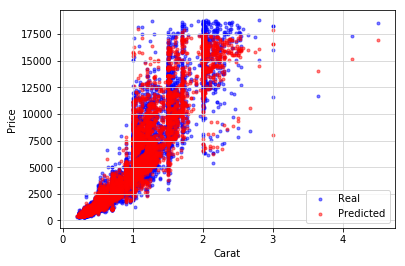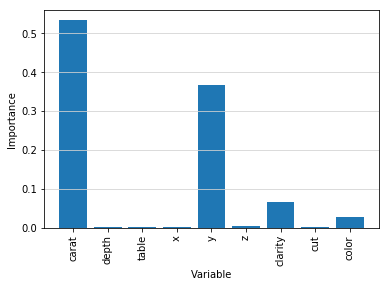# Random Forest in Python with scikit-learnThe random forest algorithm is the combination of tree predictors such that each tree depends on the values of a random vector sampled independently and with the same distribution for all trees in the forest. It can be applied to different machine learning tasks, in particular, classification and regression. Random Forest uses an ensemble of decision trees as a basis and therefore has all advantages of decision trees, such as high accuracy, easy usage, and no necessity of scaling data. Moreover, it also has a very important additional benefit, namely perseverance to overfitting (unlike simple decision tree).In this tutorial, we will use the Diamonds dataset and predict the price of the diamonds with the help of Random Forest Regressor. Then, we will visualize and analyze the obtained results. Also, we will consider the hyperparameters tuning and the importance of variables.

In :
# Import libraries
import numpy as np
import pandas as pd


Out:

Unnamed: 0 carat cut color clarity depth table price x y z
0 1 0.23 Ideal E SI2 61.5 55.0 326 3.95 3.98 2.43
1 2 0.21 Premium E SI1 59.8 61.0 326 3.89 3.84 2.31
2 3 0.23 Good E VS1 56.9 65.0 327 4.05 4.07 2.31
3 4 0.29 Premium I VS2 62.4 58.0 334 4.20 4.23 2.63
4 5 0.31 Good J SI2 63.3 58.0 335 4.34 4.35 2.75

As you can see, we have some features in the text format, and we need to encode them to the numerical format. Let's also drop the unnamed index column.

In :
# Import label encoder
from sklearn.preprocessing import LabelEncoder

diamonds = diamonds.drop(['Unnamed: 0'], axis = 1)
categorical_features = ['cut', 'color', 'clarity']
le = LabelEncoder()

# Convert the variables to numerical
for i in range(3):
new = le.fit_transform(diamonds[categorical_features[i]])
diamonds[categorical_features[i]] = new

Out:

carat cut color clarity depth table price x y z
0 0.23 2 1 3 61.5 55.0 326 3.95 3.98 2.43
1 0.21 3 1 2 59.8 61.0 326 3.89 3.84 2.31
2 0.23 1 1 4 56.9 65.0 327 4.05 4.07 2.31
3 0.29 3 5 5 62.4 58.0 334 4.20 4.23 2.63
4 0.31 1 6 3 63.3 58.0 335 4.34 4.35 2.75

As we already mentioned, one of the benefits of the Random Forest algorithm is that it doesn't require data scaling. So, to use this algorithm, we only need to define features and target.

In :
# Create features and target
X = diamonds[['carat', 'depth', 'table', 'x', 'y', 'z', 'clarity', 'cut', 'color']]
y = diamonds[['price']]


### Training the model and making prediction¶

At this point, we have to split our data into training and test sets. As a test set, we will take 25% of all data.

In :
# Make necessary imports
from sklearn.ensemble import RandomForestRegressor
from sklearn.model_selection import train_test_split

# Split data into training and test sets
X_train, X_test, y_train, y_test = train_test_split(X, y, test_size = 0.25, random_state = 101)

# Train the model
regr = RandomForestRegressor(n_estimators = 10, max_depth = 10, random_state = 101)
regr.fit(X_train, y_train.values.ravel())

Out:
RandomForestRegressor(bootstrap=True, criterion='mse', max_depth=10,
max_features='auto', max_leaf_nodes=None,
min_impurity_decrease=0.0, min_impurity_split=None,
min_samples_leaf=1, min_samples_split=2,
min_weight_fraction_leaf=0.0, n_estimators=10, n_jobs=1,
oob_score=False, random_state=101, verbose=0, warm_start=False)

Now, we have a pre-trained model and can estimate it by making the prediction of the diamonds prices and comparing them with the real prices from test data. To make this comparison more illustrative, we will show it both in the forms of table and plot.

In :
import warnings
warnings.filterwarnings('ignore')

# Make prediction
predictions = regr.predict(X_test)

result = X_test
result['price'] = y_test
result['prediction'] = predictions.tolist()

Out:

carat depth table x y z clarity cut color price prediction
46519 0.51 62.7 54.0 5.10 5.08 3.19 4 2 3 1781 1713.028900
8639 1.06 61.9 59.0 6.52 6.50 4.03 2 3 5 4452 4420.934238
23029 0.33 61.3 56.0 4.51 4.46 2.75 2 2 3 631 595.523034
51641 0.31 63.1 58.0 4.30 4.35 2.73 5 1 3 544 703.826267
25789 2.04 58.8 60.0 8.42 8.32 4.92 2 3 5 14775 15691.316331
In :
# Import library for visualization
import matplotlib.pyplot as plt

# Define x axis
x_axis = X_test.carat

# Build scatterplot
plt.scatter(x_axis, y_test, c = 'b', alpha = 0.5, marker = '.', label = 'Real')
plt.scatter(x_axis, predictions, c = 'r', alpha = 0.5, marker = '.', label = 'Predicted')
plt.xlabel('Carat')
plt.ylabel('Price')
plt.grid(color = '#D3D3D3', linestyle = 'solid')
plt.legend(loc = 'lower right')
plt.show()As you can conclude from this figure, predicted prices (red scatters) coincide well with the real ones (blue scatters), especially in the region of small carat values. But to estimate our model more precisely, we will look at Mean absolute error (MAE), Mean squared error (MSE), and R-squared scores.

In :
# Import library for metrics
from sklearn.metrics import mean_squared_error, r2_score, mean_absolute_error

# Mean absolute error (MAE)
mae = mean_absolute_error(y_test.values.ravel(), predictions)

# Mean squared error (MSE)
mse = mean_squared_error(y_test.values.ravel(), predictions)

# R-squared scores
r2 = r2_score(y_test.values.ravel(), predictions)

# Print metrics
print('Mean Absolute Error:', round(mae, 2))
print('Mean Squared Error:', round(mse, 2))
print('R-squared scores:', round(r2, 2))


Mean Absolute Error: 315.78
Mean Squared Error: 348410.39
R-squared scores: 0.98


The R-squared value is rather good, but the errors are high. To improve this situation, we should tune the hyperparameters of the algorithm a little. We can do this manually, but it will take a lot of time. Special tools from sklearn library can help us perform the tuning faster and more effective. One of such tools is GridSearchCV method which will obtain the best parameters for the algorithm.

### Tuning the parameters¶

In :
# Import GridSearchCV
from sklearn.model_selection import GridSearchCV

# Find the best parameters for the model
parameters = {
'max_depth': [70, 80, 90, 100],
'n_estimators': [900, 1000, 1100]
}
gridforest = GridSearchCV(regr, parameters, cv = 3, n_jobs = -1, verbose = 1)
gridforest.fit(X_train, y_train)
gridforest.best_params_


Fitting 3 folds for each of 12 candidates, totalling 36 fits


[Parallel(n_jobs=-1)]: Done  36 out of  36 | elapsed: 16.7min finished

Out:
{'max_depth': 70, 'n_estimators': 1100}

If you pass the obtained parameters to the algorithm, you will see that errors decreased and R-squared scores increased which means that the algorithm with the tuned hyperparameters has higher prediction accuracy.

### Defining and visualizing variables importance¶

For this algorithm, we used all the diamond features, but some of them influence the price greater than the others. If we define the most important features, we will be able to use only those in calculations and in such way improve the performance of the algorithm.

In :
# Get features list
characteristics = X.columns

In :
# Get the variables importances, sort them, and print the result
importances = list(regr.feature_importances_)
characteristics_importances = [(characteristic, round(importance, 2)) for characteristic, importance in zip(characteristics, importances)]
characteristics_importances = sorted(characteristics_importances, key = lambda x: x, reverse = True)
[print('Variable: {:20} Importance: {}'.format(*pair)) for pair in characteristics_importances];


Variable: carat                Importance: 0.53
Variable: y                    Importance: 0.37
Variable: clarity              Importance: 0.07
Variable: color                Importance: 0.03
Variable: depth                Importance: 0.0
Variable: table                Importance: 0.0
Variable: x                    Importance: 0.0
Variable: z                    Importance: 0.0
Variable: cut                  Importance: 0.0

In :
# Visualize the variables importances
plt.bar(characteristics, importances, orientation = 'vertical')
plt.xticks(rotation = 'vertical')
plt.ylabel('Importance')
plt.xlabel('Variable')
plt.grid(axis = 'y', color = '#D3D3D3', linestyle = 'solid')
plt.show()From the figure above you can see that only four features have a great influence on the prediction results. Therefore, we can use only these ones to perform the calculations.

### Conclusion¶

To sum up, we can say that the Random Forest algorithm has some advantages in comparison with Lasso, Ridge or OLS regressions. It doesn't require data scaling and has higher prediction accuracy. Random Forest algorithm is also less prone to overfitting and easier for hyperparameters tuning. Linear regression methods could be better only if you are assured that your function is linear.